# 基于 HTML5 WebGL 与 GIS 的智慧机场大数据可视化分析

2019/04/10 10:10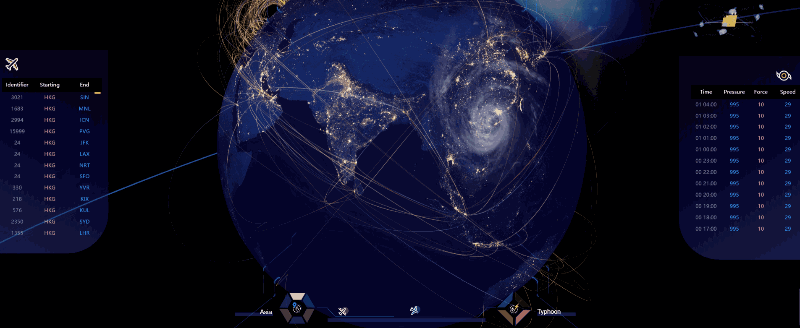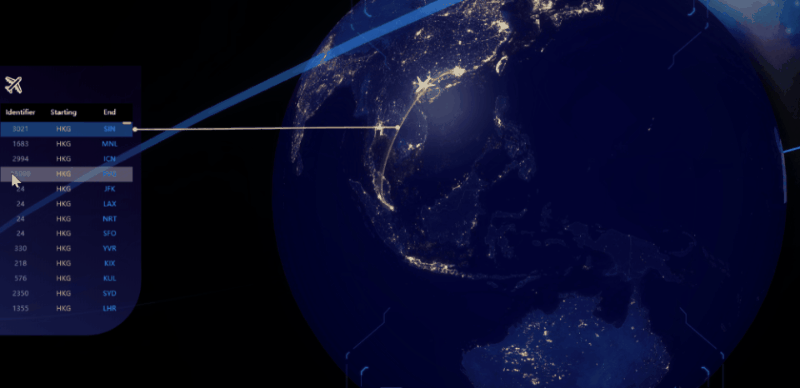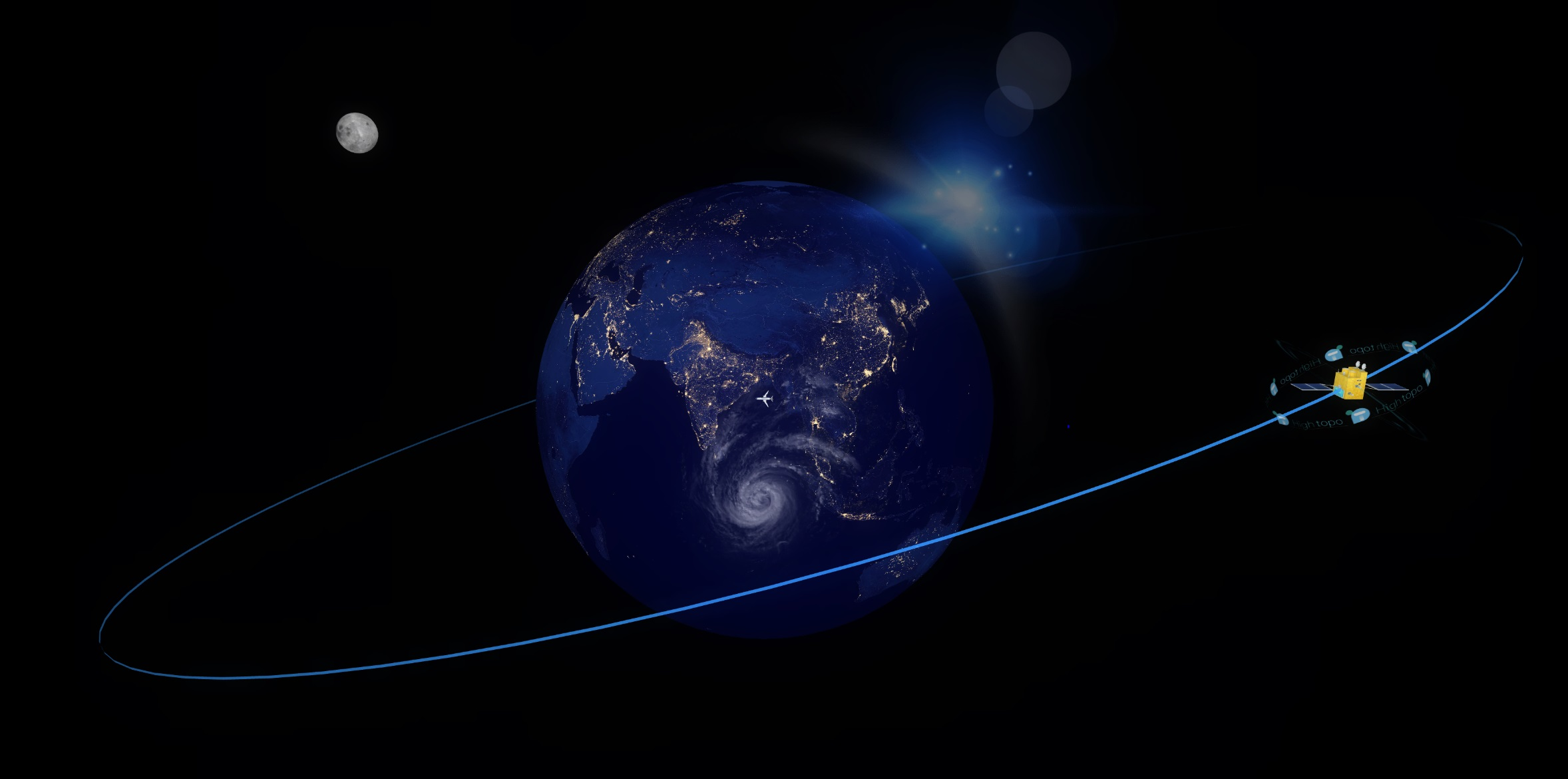2D 面板部分主要包括左侧航线表格，右侧风暴实时数据表格以及底部的信息面板。左侧航线表格展示了不同大洲的航线信息，大洲可以通过底部的左侧按钮进行切换；右测风暴信息是模拟生成，实时更新；底部信息栏包括大洲按钮及航线详细信息。面板截图：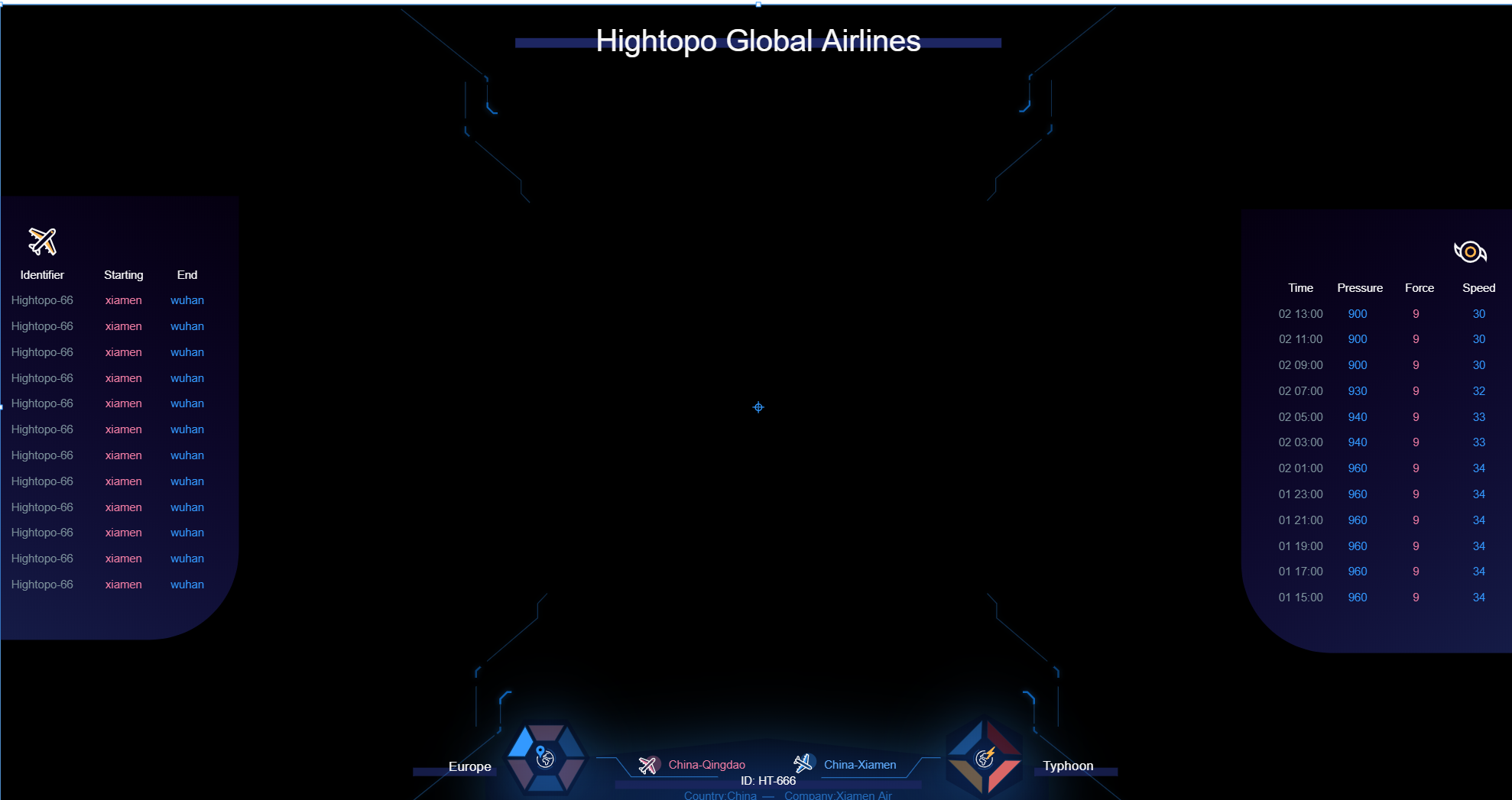[[-9.443380356,147.2200012, 146,"POM","OC","PG","PG-NCD","Port Moresby"],
[63.98500061,-22.60560036, 171,"KEF","EU","IS","IS-2","Reykjavík"],
[36.001741,117.63201,0,"CN-0083","AS","CN","CN-U-A",""],
…
]

{"MIA":["3201:PUJ","24:MSY","24:MVD","24:NAS","24:ORF","24:PHL","24:PTP","24:PTY","24:RIC","24:SAL","24:SAN","24:SDQ","24:SFO","1299:AMS"],
"HKG":["3021:SIN","1683:MNL","2994:ICN","15999:PVG","24:JFK","24:LAX","24:NRT","24:SFO","330:YVR","218:KIX","576:KUL","1680:SGN","328:POM"],
"SJU":["3029:SXM","3029:TPA"],
…
}

// 将经纬度转换为球体位置
getSpherePos(radius, longitude, latitude) {
let ang1 = Math.PI * (longitude - 90) / 180;
let ang2 = Math.PI * latitude / 180; let x, y, z; let s_r = radius; x = s_r * Math.sin(ang1) * Math.cos(ang2); y = s_r * Math.cos(ang1) * Math.cos(ang2); z = s_r * Math.sin(ang2); return [x, y, z]; }

/**
* 根据航线起点，终点位置创建航线（贝塞尔曲线）
* @param {Object} start 起点机场信息
* @param {Object} end 终点机场信息
*/
createEdge(start, end) {
let edge;
let distance = ht.Default.getDistance(start.point, end.point); let ratio = distance / this.radius; let v1 = new ht.Math.Vector3(start.point); let v2 = new ht.Math.Vector3(end.point); let v3 = v1.clone().add(v2).setLength(distance / 2); let v4 = v3.clone().add(v2); v3.add(v1); ​ edge = new ht.Polyline(); ​ // 此处设置 edge 样式和属性的代码省略  edge.setPoints([ { x: start.point, y: start.point, e: start.point }, { x: v3.x, y: v3.z, e: v3.y }, { x: v4.x, y: v4.z, e: v4.y }, { x: end.point, y: end.point, e: end.point }, ]); edge.setSegments([1, 4]); this.dm3d.add(edge); edge.setHost(this.earth); }

这部分的难点在于如何根据航线的起点和终点位置构造中间控制点来生成贝塞尔曲线。下面的示意图演示了代码中向量的计算及各个向量变量的变化。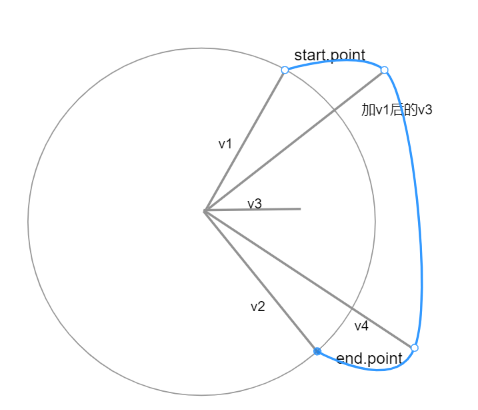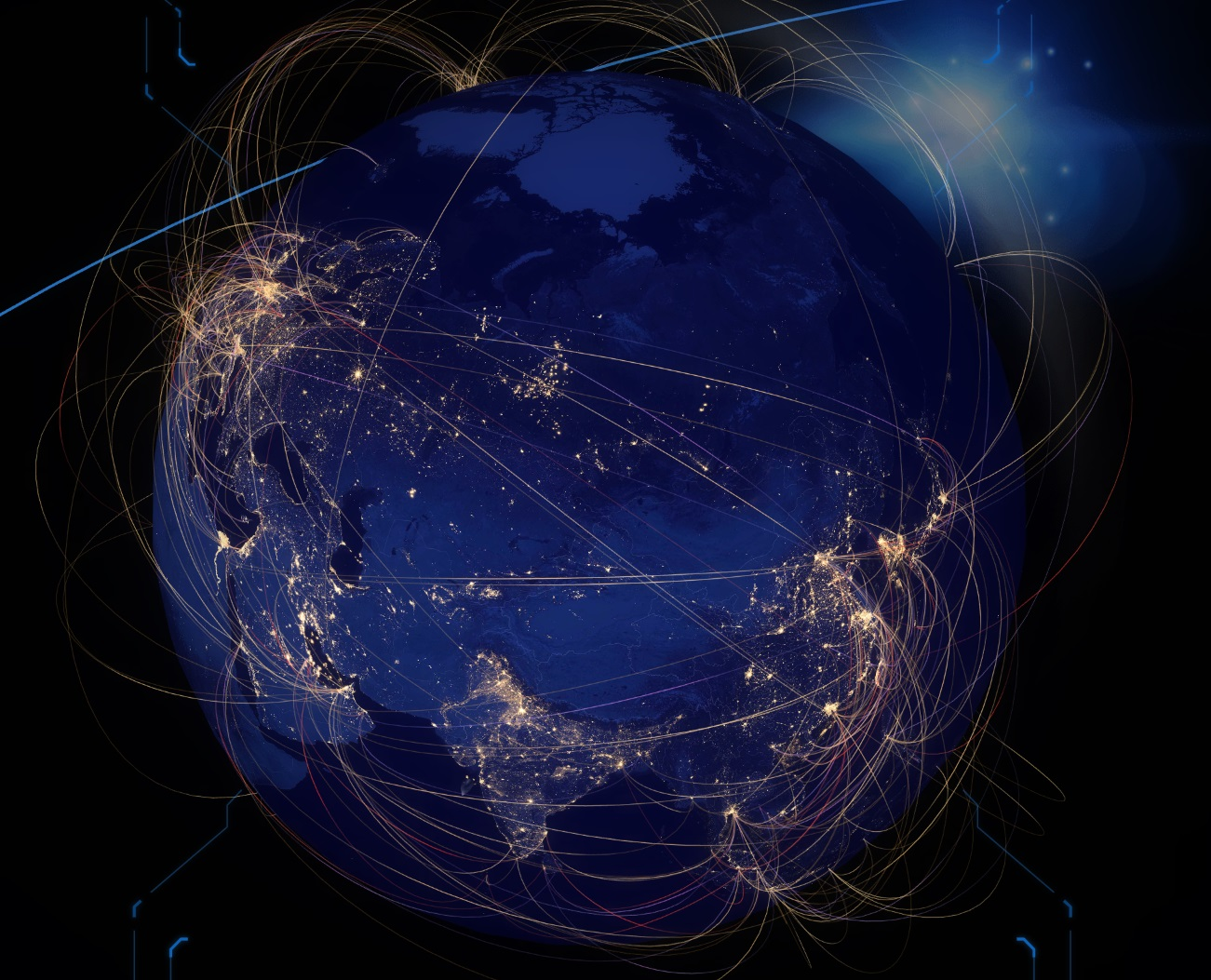2D/3D 互动画线

// 获取定位线的终点 -- 3D 球体中选中航线对应的位置
getLineEnd() {
let p3 = this.g3d.getLineOffset(this.selectedEdge, this.g3d.getLineLength(this.selectedEdge) * 0.5); let p = g3d.toViewPosition([p3.point.x, p3.point.y, p3.point.z]); p = this.g2d.getLogicalPoint(p); this.endPoint = p; }

// 获取定位线的起点 -- 航线表格对应的位置
getLineStart() {
let offset = this.table.a('ht.translateY'); let lineStartPoint = {}; let height = this.table.getHeight(); let origY = this.table.p().y - height / 2 + this.table.a('ht.headHeight') + this.table.a("ht.rowHeight") / 2; lineStartPoint.x = this.table.p().x + this.table.getWidth() / 2; lineStartPoint.y = origY + this.rowIndex * this.table.a("ht.rowHeight") + offset; this.startPoint = lineStartPoint; }

ht.Default.startAnim({
frames: 60,
interval: 16,
finishFunc: function() { console.log('finish'); }, action: function(t) { console.log(t); } });

action: function (v, t) {
let offset = that.g3d.getLineOffset(that.selectedEdge, length * v); // 偏移量
let p1 = offset.point; // 3D 坐标 let tangent = offset.tangent; // 切线方向 let direction = new ht.Math.Vector3(tangent); let vp1 = new ht.Math.Vector3(p1); direction.multiplyScalar(0.1); direction.add(vp1); direction.setLength(direction.length() + 2); vp1.setLength(vp1.length() + 2); that.airPlane.p3(vp1.x, vp1.y, vp1.z); that.airPlane.setRotationMode('yxz'); that.airPlane.lookAtX([0, 0, 0], 'bottom'); that.airPlane.lookAtX([direction.x, direction.y, direction.z], 'front'); lightP = new ht.Math.Vector3(p1); lightP.setLength(that.radius * 2); that.spotLight.p3(lightP.x, lightP.y, lightP.z); direction.setLength(that.radius); lightP.setLength(that.radius); that.planeShadow.p3(lightP.x, lightP.y, lightP.z); that.planeShadow.setRotationMode('yxz'); that.planeShadow.lookAtX([0, 0, 0], 'back'); that.planeShadow.lookAtX([direction.x, direction.y, direction.z], 'right'); }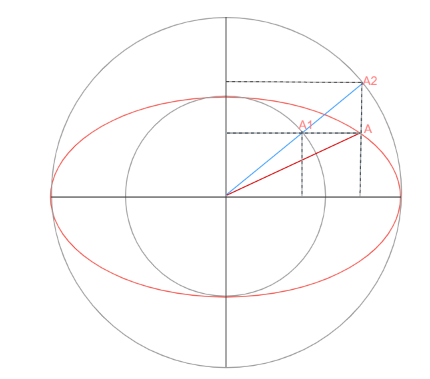Logo 和光晕的旋转使用了 3D 旋转函数，具体使用方法可以参照  HT 3D 手册 中的 3D 旋转函数部分。卫星动画的代码实现如下所示：

// 卫星及 Logo 的旋转
startSat() {
let dm = this.dm3d; let a = 1226; // 椭圆半长轴 let b = 698; // 椭圆半短轴  let x, y, z; y = 0; let sat_ang = 0; // 卫星初始角度 let logo_ang = 0; // Logo 初始角度 setInterval(() => { sat_ang = sat_ang + this.satelliteSpeed; logo_ang = logo_ang + 0.01 x = a * Math.cos(-sat_ang); // 卫星当前 x 轴坐标 z = b * Math.sin(-sat_ang); // 卫星当前 z 轴坐标 y = x * Math.sin(Math.PI * 16 / 180); // 卫星当前 y 轴坐标 x = x * Math.cos(Math.PI * 16 / 180); // 卫星轨道面沿 z 轴旋转之后的新的 x 轴坐标 this.sat.p3(x, y, z); this.logo.setRotationY(logo_ang); this.logo.setRotationZ(28 / 180 * Math.PI); this.logo.setRotationMode('yzx'); this.sat_p.setRotationY(logo_ang); this.sat_p.setRotationZ(-35 / 180 * Math.PI); this.sat_p.setRotationMode('yzx'); }, 16.7); }

// 风暴动画
startStorm() {
let s_ang = 0;
let s_ang2 = 0; let s_x, s_y, s_z; let s_r = 380.07; setInterval(() => { s_ang = s_ang + 0.002; s_ang2 = s_ang2 + 0.002; s_x = s_r * Math.sin(s_ang) * Math.cos(s_ang2); s_z = s_r * Math.cos(s_ang) * Math.cos(s_ang2); s_y = s_r * Math.sin(s_ang2); this.storm.p3(s_x, s_y, s_z); this.storm.lookAtX([0, 0, 0], 'bottom'); this.storm.setRotationMode('yzx'); this.storm.setRotationY(s_ang * 20); }, 60); }

this.maxDisplayCount = 300; // 30s 更新一次航线
this.MAX_DISPLAY_COUNT = 6; edge.s({ // 创建航线时 'display_flag': parseInt(Math.random() * 10) % this.MAX_DISPLAY_COUNT, }); start() { this.edgeTimer = setInterval(() => { this.edges.forEach((val) => { let showFlag = this.checkStormDistance(val); showFlag = showFlag && (val.s('display_flag') == this.displayFlag); val.s('3d.visible', showFlag) }); this.displayCount++; if (this.displayCount > this.maxDisplayCount) { this.displayFlag = (this.displayFlag + 1) % this.MAX_DISPLAY_COUNT; this.displayCount = 0; } }, 100); }

- Polyline resolution 动态改变

HT 通过微分段的方式实现曲线，参数 shape3d.resolution 用来控制曲线微分段数，这个参数决定 3D 图形精度，数值越大曲线越均匀，但同时会影响性能。在本 Demo 中，为防止飞机抖动 shape3d.resolution 设置为 60。但是这样设置之后，性能影响会很大，因此我们采用了动态调整 resolution 的方式，根据航线是否被选中动态调整，提高性能。代码如下。在 updateResolution 中也需要调用 g3d.invalidateCachedGeometry(data) 来重置 geometry，更新方法见 “Polyline cache 以及更新方法” 部分。

// 动态改变 resolution
updateResolution(isRestore) {
if (!this.selectedEdge) { // 没有航线被选中 return; } let res, thickness; let len = this.g3d.getLineLength(this.selectedEdge); if (isRestore) { // 需要恢复默认值 res = 30; thickness = 0.7; } else { res = len / 200 * 30; if (res < 60) { res = 60; } thickness = 5; } this.selectedEdge.s('shape3d.resolution', res); this.selectedEdge.setThickness(thickness); }

- Polyline cache 以及更新方法

// 创建航线时设置属性
edge.s({
'geometry.cache': true }); // this.selectedEdge 属性发生变化时，重置 geometry。 let ui = g3d.getData3dUI(this.selectedEdge); ui.shapeModel = ui.info = null; this.g3d.invalidateData(this.selectedEdge);

- 2D/3D 互动画线调用 setTimeout

this.updateTimer = setTimeout(() => {
this.updateTimer = null; if (this.selectedEdge == null) { // 没有航线被选中 return; } this.getLineEnd(); // 计算 2D/3D 定位线的终点 this.updateLine(true); // 绘制定位线 }, 50);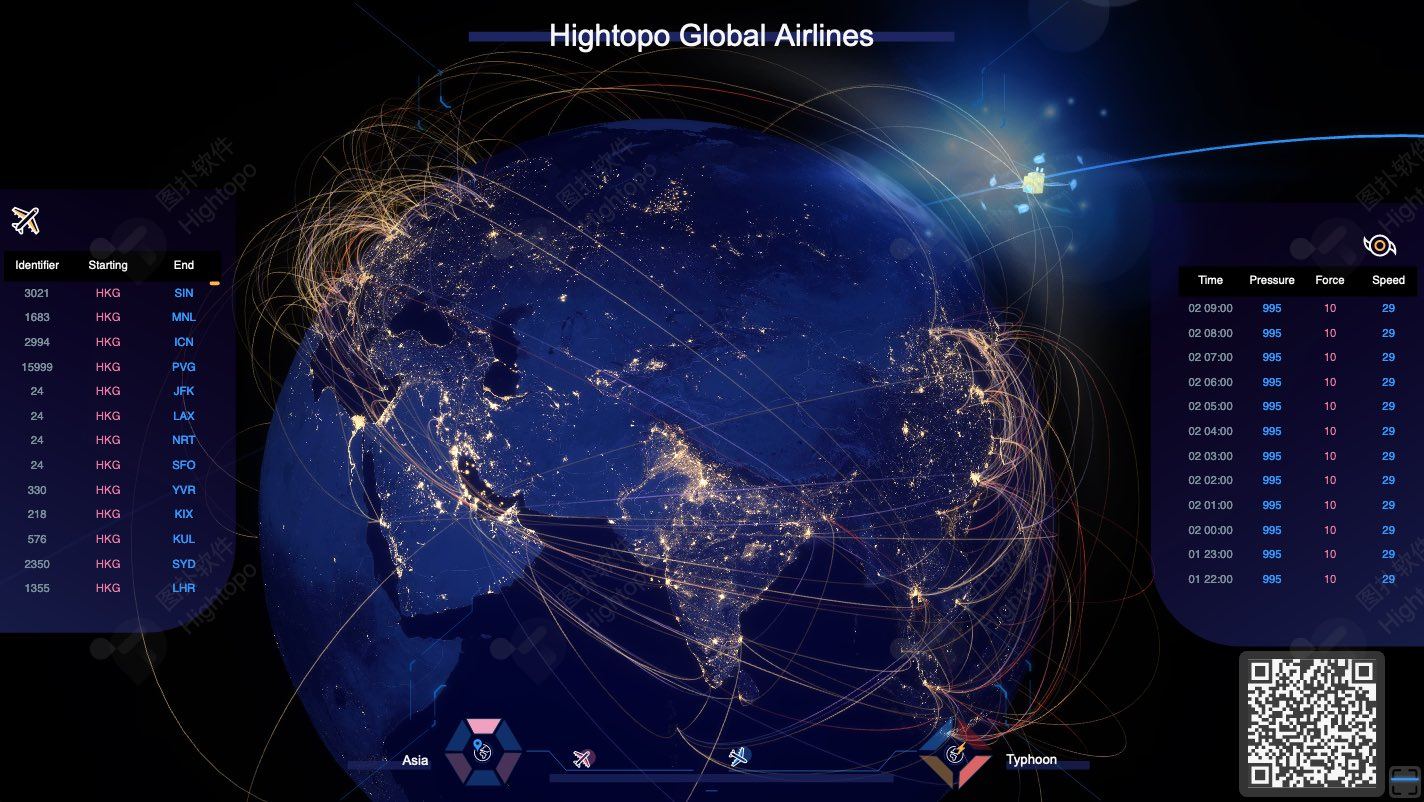0
0 收藏

### 作者的其它热门文章0 评论
0 收藏
0